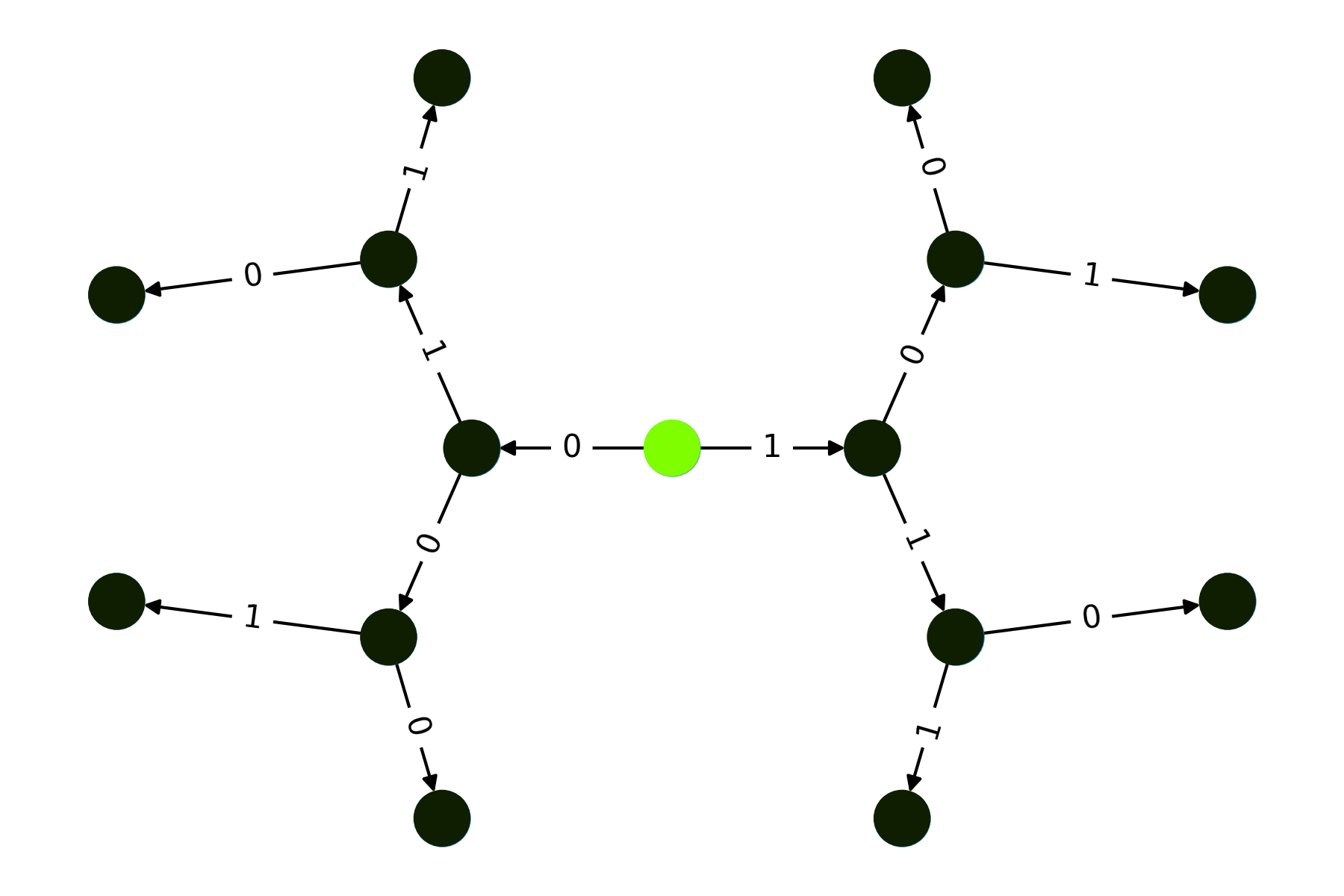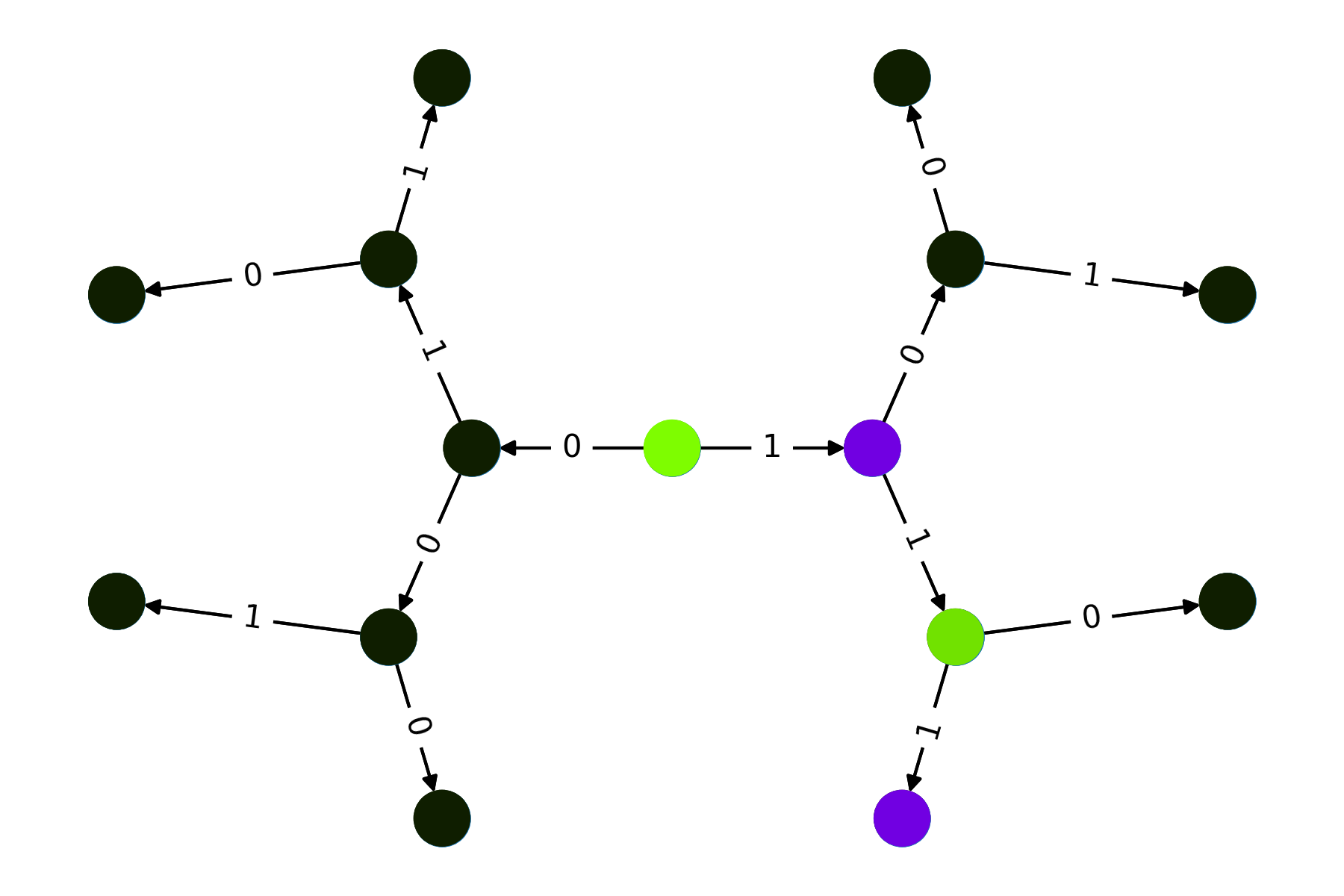# qrisp.quantum_backtracking.QuantumBacktrackingTree.visualize_statevector#

QuantumBacktrackingTree.visualize_statevector(pos=None)[source]#

Visualizes the statevector graph.

Parameters
posdict, optional

Examples

We initialize a backtracking tree and visualize:

```from qrisp import auto_uncompute, QuantumBool, QuantumFloat
from qrisp.quantum_backtracking import QuantumBacktrackingTree
import matplotlib.pyplot as plt

@auto_uncompute
def reject(tree):
return QuantumBool()

@auto_uncompute
def accept(tree):
return (tree.branch_qa == 1) & (tree.branch_qa == 1) & (tree.branch_qa == 1)
```
```>>> tree = QuantumBacktrackingTree(3, QuantumFloat(1, name = "branch_qf*"), accept, reject)
>>> tree.init_node([])
>>> tree.visualize_statevector()
>>> plt.show()
``````>>> tree = tree.copy()
>>> tree.init_phi([1,1,1])
>>> tree.visualize_statevector()
```